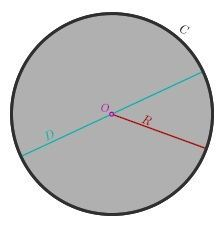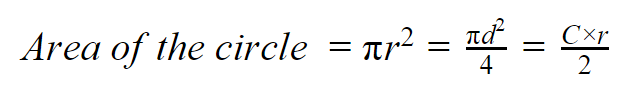# Area of a Circle Formula

A circle is a round figure that consists of points equidistant from a certain or fixed point to the central point of the circle. The central point from where the radius is drawn is known as the centre of the circle. The radius of a circle is the distance from the centre of a circle to any point on the circle. If you place two radii end-to-end in a circle, you would have the same length as one diameter. Thus, the diameter of a circle is twice as long as the radius.  The area of a circle is the number of square units inside that circle. A circle has the maximum possible area for a given perimeter and the minimum possible perimeter for a given area. The perimeter C of a circle is called the circumference.The Area of a Circle Formula is given as,Where
r is the radius of the circle.
d is the diameter of the circle.
C is the circumference of the circle.

### Solved Example

Question 1: Find the area of the circle whose radius is 8 cm.
Solution:

Given,
Radius of a circle = r = 8 cm
Area of a circle
=
$$\begin{array}{l}\pi\end{array}$$
r2
=
$$\begin{array}{l}\pi\end{array}$$
$$\begin{array}{l}\times\end{array}$$
82 cm2
= 3.14 × 64 cm2
= 200.96 cm2